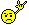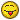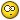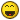# A Simple Sinewave Generator

I think it would be good to just have a very basic sinewave synth inside renoise, nothing more. it would just be a rightclick-menu job in the sample slot, so it would just come up as ‘sinewave’ and you wouldn’t see a waveform or anything in the sample edit window.

i don’t want renoise turning into a soft-studio with built in synths to make it weaker but i do find my self wasting time looking for my .wav sinewave sample, i have a massive HD crammed with .wavs i don’t know where to look, and my VSTI list is huge i can never remember which waveform options each vsti has.

good idea yes? instant sub bass!!!EXPANDING ON THIS IDEA:

Quick context menu on right click in sample editor? Choose from Sine/Saw/Square/Pulse/etc? Maybe allow for custom waveforms? Insta-chip :3

Or, why not just make a waveform drawing tool?

+1

Suggested many times before in many ways…

+65367587666554675454344567e92

holy shit +7

this would seriously be wonderful.

you could make limitless sounds and instruments, the sky, the sky! is the limit!

pleeeeeeeeeeeeeeeeease implement internal waveform generation.

+1

with emphasis on not turning it into a built-in synth!

if nothing else, you could use it as a tone reference tool when trying to figure out what to cut and where when EQing.

i, of course would be using it for SUB BASS!In zero x beatquantizer ( which is a standalone beatslicer) there is a tool called ‘formula waveshaper’. This is a handy utility in which you can generate different waveforms according to formula’s. There are a lot of preset formula’s for one to choose from, like:

Formula=“sin(x)”

Formula=“sin(x)sin(x2)”

Formula=“arctan(tan(x))”

Formula=“arctan(tan(x))*sin(x)”

Formula=“arctan(tan(x))*cos(x)”

Formula=“rand(x)”

Formula=abs(x-P)-P/2-sin(x)

Formula=sin(x)+sin(x2)+sin(2P-x3)+sin(4x)+sin(2P-6x)

Formula=x-P-sin(x)

Formula=sin(x/4)-P/6

Formula=sin(int(x*15/P)*P/15)

Formula=sin(sin(sin(sin(sin(sin(sin(sin(sin(sin(sin(sin(sin(sin(sin(sin(sin(sin(x))))))))))))))))))

Formula=arctan(tan(x))+arctan(tan(x/2))

Formula=x-P

Formula=arctan(tan(x))*cos(x/4)round(arctan(x8))

Formula=arctan(tan(x))*cos(x/4)round(arctan(x8))

Formula=sin(x*3)/tan(x)*sin(x/2)

Formula=sin(x*3)/tan(x)*sin(x/2)

Formula=tan(sin(x))/arctan(sin(x/5)/cos(x*50))

Formula=sin(x)*100

Formula=sin(x)+sin(x2)+sin(x3)

Formula=sin(x)+sin(x2)+sin(x3)+sin(x4)+sin(x5)

Formula=sin(int(x/P)*P+P/2)

Formula=sin(x)^11

Formula=int(P^19*sin(x)^3)

Formula=sin(x)^5+cos(x)^5

Formula=(abs(sin(x/2))-abs(cos(x/2)))^3

Formula=abs(x-P)-P/2

Formula=x*(1-0.2xx)

Formula=cos(sinh(x*3))

Maybe interesting if this ever gets implemented.

@jonas the plugexpert:
Please don’t make things complex dude, it’s been about two years that we cry for a simple sin(x), now you expect (abs(sin(x/2))-abs(cos(x/2)))^3 ?where can i find a library of simple waveforms?

http://www.galbanum.com/products/architecture-universal/

• “Architecture Universal Waveforms, is a collection of over 1,800 meticulously designed wavetables provided in industry standard .wav format. Wavetables are single cycle periodic waveforms that form the most fundamental building block of synthesis. They are the sound generators, and can be thought of as the oscillator of a classic analog synth, but they provide infinitely more variability in shape and spectrum. They are chiefly responsible for determining a given sound’s primary timbre, or “tone color”. This collection offers a huge variety of waveforms logically organized in sub-folder categories.”

last i checked (08-26-07) > \$30

edit: if you plan on using them, be wary of dangerous looking high frequency waveforms > make sure the volume is low, HF waveforms equates to accidentally pushing the resonance all the way up, causing that needle piercing soundwave > that shit ƒuck!n hurts

Take them from good quality vst demosGimme a simple waveform editor, very basic.
I’m going to try out the Angular Momentum Freehand, thx for the tip.

+1 … whatever it may means

Yeah this is needed.

Just something basic in the sampler.

Sine generator and a pitching tool with adjustable semi-tones (like the synth engine in Sound Forge).

Sub bass and Kick drums could be synthed in house. Nice.

-1

If you want something that INSTANT, then just download AutoHotKey or any other macro-making software and create a custom macro that loads the sine.wav from a given directory on your HD (or at least opens the directory with different waveforms for you to select from) once you press the assigned key.

http://www.autohotkey.com/

Yeah, I agree. No need to clutter up the rns interface with fluff. Just make your own library of basic waveforms. I use audition > generate tones for that.

-1

formula to sample/envelopes ain’t fluff!.. and could simply be added to the context menu, no clutter required.

Bloat, then.

it wouldn’t increase the exceutable size in any meaningful way, it wouldn’t slow down any operations not using it, it wouldn’t take up any screen estate… bloat??how much does adobe audition weigh? how much would a function to turn forumulas into samples/envelopes weigh? you’re kidding, right?

This to me is an excellent idea. Seems pretty old school and wicked. It is the kind of thing I would run into when I was a kid, messing with old audio software and think, “what is this for? it seems dark and interesting”

I say, include it. It would only need to be a case of right clicking on a blank area in a fresh instrument in the sample editor, and selecting Generate > Wave > Sine. A dialog would appear and you can select the length and frequency

Simple.

Then add Pitch bending capabilities like in Sound Forge and we are laughing! Bye Bye external editor!!!